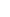19. IMAGE AND SOURCE AND THE 44TH BITLets see the topic Image and Source here by going back to the example in the 44th Bit and using real values. Lets find where Image Objects of planes are relative to the transmitters reference system at the moment Source Objects of the planes reach the meeting line.We will make use of Alice Equations for the calculation.Now that we examined the event for an observer at the Signal Station (and this observer can be a radar, as well), this time the signal transmitter is the planes. The signal station is now in the position of the signal receiver. In the equation above, lets find the values of numerator and denominator respectively:The distance the OUTGOING signal covers relative to the transmitter: We know that the locations of Source Objects of planes are at the meeting line. The distance between the meeting line and the Signal Station gives us the distance the OUTGOING signal covers relative to the transmitter.Speed of the OUTGOING signal relative to the transmitter: We already calculated the signal speeds in the part Byte Shift. If the signal goes from the Signal Station to the plane at the top at c+v speed, the speed of the signal going to the signal station from the plane is (c+v), as well. Similarly, the speed of the signal going to the station for the plane at the bottom is (c-v). We already calculated the signal speed. So, there is nothing unknown in the topic speed of the OUTGOING signal relative to the transmitter.Speed of the INCOMING signal relative to the receiver: There is no need to calculate it as it is always c.The distance the INCOMING signal covers relative to the receiver: This is a value that we need to find. The coordinate value of the emitted signal at relative to the receivers reference system gives the distance at which it will see the image object. When we calculate this value for both the planes, we find the locations of planes Image Objects.We see the necessary calculation in the table below. The values that are found were placed beforehand while preparing the figure above.Now, lets find which value for the distance of the Signal Station from them the chief pilots in the cockpit will see when they look at the radar screen.Now that we know the locations of the planes, the Signal Station, and Image Objects of the planes, we can make use of the parallelogram method. But we have a tiny problem. As the movements take place on the X axis, how can we form the parallelogram? There is an easy way. Lets form the parallelograms first and then carry the Objects to the X axis. After seeing the locations of objects on the X axis, we can show where image objects of the Signal Station relative to the planes by making use of the values in the table. We see these processes in the figure below.We get a good result from here. The location of the image object of an object can be at different locations relative to different reference systems.